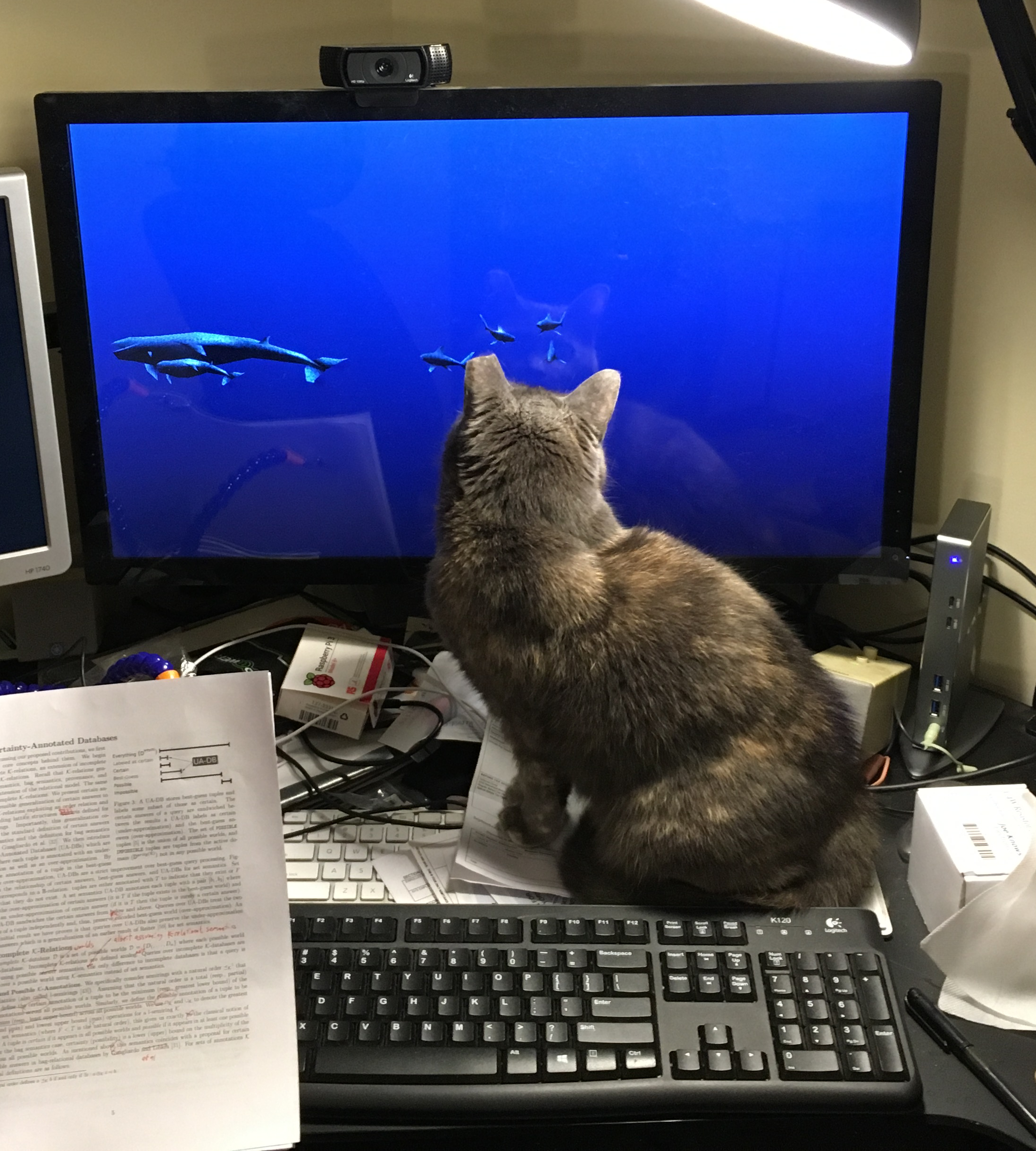CSE-250 Fall 2022 - Section B - Function Analysis

### Function Analysis

Sept 7, 2022

#### Announcements

• AI Assignment Due TONIGHT.
• PA 0 due Friday Night (68%/90% progress towards early PA1 submissions).
• Office Hour locations posted.

## What is "Fast"?

#### When is an algorithm "fast"?

• Real world ("Wall Clock") time?
Is 10s fast? 100ms? 10μs?
Do you rank the algorithm or the implementation?
Compare Grace Hopper's implementation to yours.
CPU Effects (e.g., ARM RK3399S vs Intel i9 vs AMD 5950)
Bottlenecks: CPU vs IO vs Memory vs Network vs ...
• Wall-clock time is not great for a 50k-ft view.

(a) and (b) are more "the same" than either is to (c)

#### When is an algorithm "fast"?

• Look at relative performance.
• How does performance change relative to...
• ... the number of input records
• ... the number of users in the system
• ... the number of pixels to be displayed
• ... the size of the neural network

Idea: Classify runtimes by the "shape" of their scaling curve.

#### Scaling

"Five steps plus ten steps per user"
$5 + 10 \times |\texttt{Users}|$
"Ten steps per connection; Each user has connections to 1% of the other users"
$10 \times ( |\texttt{Users}| \times (0.01 \times |\texttt{Users}|))$
"Seven steps for every possible combination of users"
$7 \times (2^{|\texttt{Users}|})$
"For each user: ten steps plus three per post"
$|\texttt{Users}| \times (10 + 3 \times |\texttt{Posts}|)$

#### When is an algorithm "fast"?

Focus on how the algorithm scales.
Look at the number of steps as a function of "input size".
,1,2,3,4,5,6,7 3 x |Users| + 5,8,11,14,17,20,23,26 |Users| x |Users|,1,4,9,16,25,36,49

Which is better $3|\texttt{Users}|+5$ or $|\texttt{Users}|^2$?

#### When is an algorithm "fast"?

Focus on how the algorithm scales.
Look at the number of steps as a function of "input size".
Focus on "large" inputs.
Look at the number of steps on large input sizes.

#### What hardware are you using?Intel i9 vsMotorola 68000

#### Who implemented the algorithm?Epic-Level Implementation vsError 23: Cat on Keyboard

#### Goal: Judge Algorithms, Not Implementations

How fast is a step?
Don't care, only count number of steps
Can we cut out steps?
Don't care...

#### When is an algorithm "fast"?

Focus on how the algorithm scales.
Look at the number of steps as a function of "input size".
Focus on "large" inputs.
Look at the number of steps on large input sizes.
Focus on high-level ideas, not implementation.
???

... a brief digression ...

## Logarithms

### (Refresher)

#### Logarithms (Refresher)

• Let $a, b, c, n > 0$
• Exponent Rule: $\log(n^a) = a \log(n)$
• Product Rule: $\log(an) = \log(a) + \log(n)$
• Division Rule: $\log\left(\frac{n}{a}\right) = \log(n) - \log(a)$
• Change of Base from $b$ to $c$: $\log_b(n) = \frac{\log_c(n)}{\log_c(b)}$
• Log/Exponent are Inverses: $b^{\log_b(n)} = \log_b(b^n) = n$

#### Logarithms (Refresher)

In this class, assume base-$2$ logarithms by default.

Question: How do we talk about runtime?

#### Attempt 1: Growth Functions

$$f(n)$$
$n$: The "size" of the input
e.g., the number of users, rows of data, etc...
$f(n)$: The number of "steps" taken for an input of size $n$
e.g., 20 steps per user is $20\times n$ (with $n = |\texttt{Users}|$)

Side Note: A growth function is not code; it's an algebraic function.

#### Attempt 1: Growth Functions

Assumptions about $f(n)$

Problem sizes are non-negative integers
$n \in \mathbb Z^+ \cup \{0\}$
We can't reverse time
$f(n) \geq 0$
Smaller problems aren't harder than bigger problems
For any $n_1 < n_2$, $f(n_1) \leq f(n_2)$

To make the math simpler, we'll allow fractional steps.

#### Attempt 1: Growth Functions

$$f : \mathbb Z^+ \cup \{0\} \rightarrow \mathbb R^+$$

Problem: We're still implementation dependent...

$$f_1(n) = 20n$$ $$f_2(n) = 19n$$
$$f_3(n) = \frac{n^2}{2}$$

$f_1$ and $f_2$ are more "the same" than either is to $f_3$

#### Behavior At Scale

Compare the following two functions: $$f_1(n) = \frac{1}{100}n^3 + 10n + 1000000\log(n)$$ $$f_2(n) = n^3$$

#### Behavior At Scale

,1,2,4,8,16,32,64,128,256,512,1024,2048,4096,8192,16384,32768 f1,10.01,1000020.08,2000040.64,3000085.12,4000200.96,5000647.68,6003261.44,7022251.52,8170332.16,10347297.28,20747658.24,96919825.92,699235727.36,5510640058.88,43994628951.04,351859048568.32 f2,1,8,64,512,4096,32768,262144,2097152,16777216,134217728,1073741824,8589934592,68719476736,549755813888,4398046511104,35184372088832

After ~1024, both lines stay ~100x apart.

$f_1$ and $f_2$ "behave" the same.

#### Behavior At Scale

$$\lim_{n\rightarrow \infty}\frac{\frac{1}{100}n^3+10n+1000000\log(n)}{n^3}$$
$$=\lim_{n\rightarrow \infty}\frac{\frac{1}{100}n^3}{n^3}+\frac{10n}{n^3}+\frac{1000000\log(n)}{n^3}$$
$$=\lim_{n\rightarrow \infty}\frac{1}{100}+\frac{10}{n^2}+\frac{1000000\log(n)}{n^3}$$
$$=\frac{1}{100} + 0 + 0$$

### Attempt 2: Asymptotic Analysis

#### Asymptotic Analysis @ 5000 feet

Consider $f(x)$ vs $g(x)$

Case 1: $lim_{n\rightarrow \infty}\frac{f(n)}{g(n)} = \infty$

($f(n)$ gets bigger w.r.t. $n$)

If $f(n)$, $g(n)$ are the number of steps an algorithm takes on an input of size $n$, which is better?

#### Asymptotic Analysis @ 5000 feet

Case 1: $lim_{n\rightarrow \infty}\frac{f(n)}{g(n)} = \infty$

($f(n)$ is "bigger"; $g(n)$ is the better runtime on larger data)

Case 2: $lim_{n\rightarrow \infty}\frac{f(n)}{g(n)} = 0$

($g(n)$ is "bigger"; $f(n)$ is the better runtime on larger data)

Case 3: $lim_{n\rightarrow \infty}\frac{f(n)}{g(n)} = \text{some constant}$

($f(n)$, $g(n)$ "behave the same" on larger data)

#### Asymptotic Analysis @ 5000 feet

Goal: Organize runtimes (growth functions) into different Complexity Classes.

Within a complexity class, runtimes "behave the same"

#### Asymptotic Analysis @ 5000 feet

"Strategic Optimization" focuses on improving the complexity class of code.

#### Asymptotic Analysis @ 5000 feet

$$f_1(n) = \frac{1}{100}n^3 + 10n + 1000000\log(n)$$ $$f_2(n) = n^3$$

The $10n$ and $1000000\log(n)$ "don't matter"

The $\frac{1}{100}$ "doesn't matter"

The $n^3$ "dominates" both growth functions for large $n$.

#### Asymptotic Analysis @ 5000 feet

$5\cdot 2^n + n^{1000} + 2^{\log(n)}$

#### Why focus on dominating terms?

$f(n)$ 10 20 50 100 1000
$\log(\log(n))$ 0.43 ns 0.52 ns 0.62 ns 0.68 ns 0.82 ns
$\log(n)$ 0.83 ns 1.01 ns 1.41 ns 1.66 ns 2.49 ns
$n$ 2.5 ns 5 ns 12.5 ns 25 ns 0.25 µs
$n\log(n)$ 8.3 ns 22 ns 71 ns 0.17 µs 2.49 µs
$n^2$ 25 ns 0.1 µs 0.63 µs 2.5 µs 0.25 ms
$n^5$ 25 µs 0.8 ms 78 ms 2.5 s 2.9 days
$2^n$ 0.25 µs 0.26 ms 3.26 days 1013 years 10284 years
$n!$ 0.91 ms 19 years 1047 years 10141 years 🤯
$$2^n \gg n^c \gg n \gg \log(n) \gg c$$

#### Next time on CSE-250

Asymptotic notation: This time it's formal